# Using Python and openCV to extract and manipulate plot lines

Someone on the PLOTS-spectrometry mailing list posted two spectrographs they'd collected with their PLOTS-spectrometer, and also a plot the LED manufacturer provided.
The wondered why their spectrogram didn't look like either of the two plots provided by the manufacturer, and I thought they might look similar if the plot lines were added or multiplied with each other.
So I made this script to select the plot line color, then extract colors close to that into separate images.
With these images, the maximum Y value for each column was found, and Y values from the corresponding plots were added and also multiplied, then saved to new output images.

## LED spectrograms in question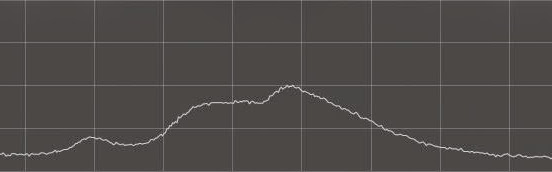## Using this dual-line graph as input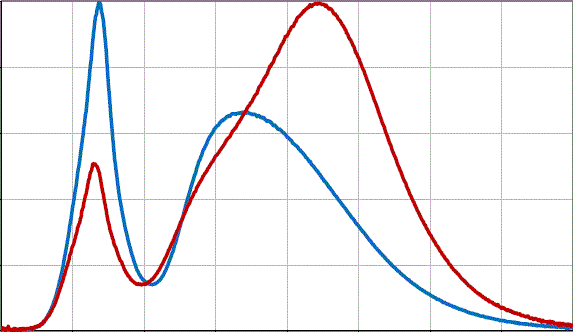## These binary images were extracted of the individual plot lines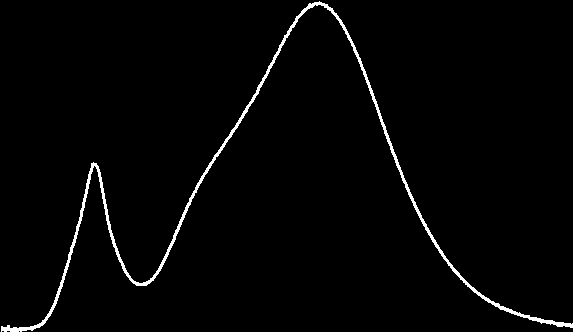## Plots' Y-values added together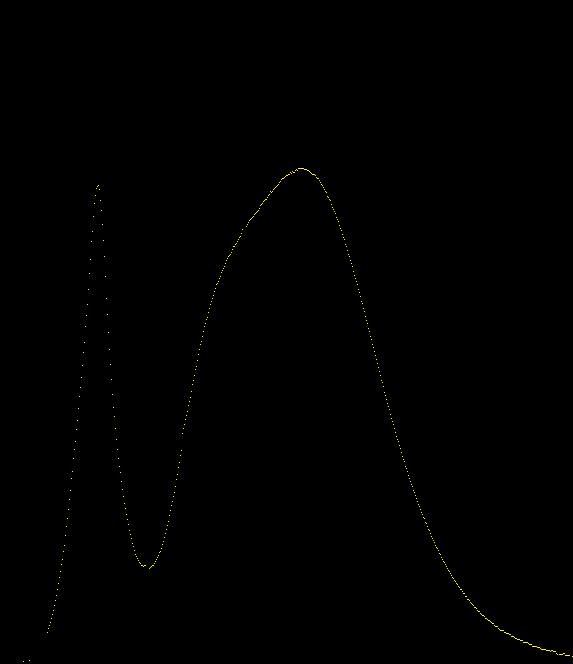## Added, then divided by 2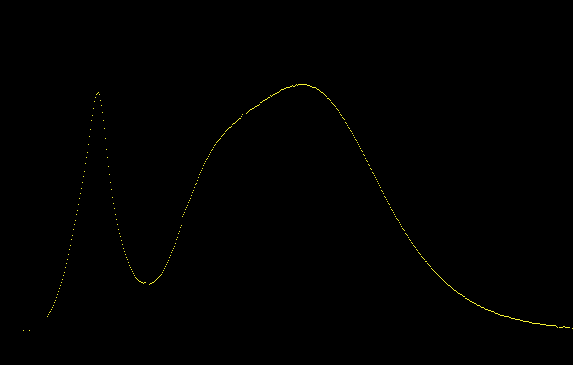## Square root of the multiples ( sqrt(y1 * y2) )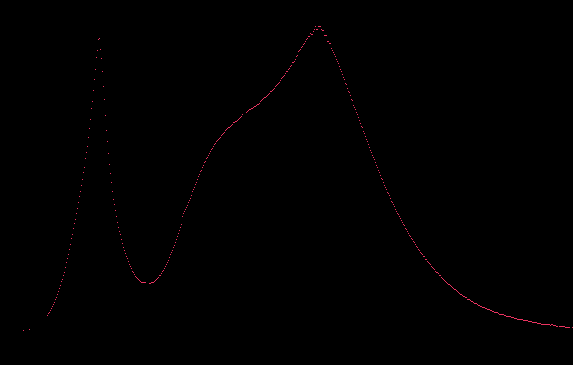## Graph of added (yellow) and multiplied (red) lines, both were scaled down.## Using this triple-line graph image as input## Binary images of extracted plots## Added## Added then divided by 3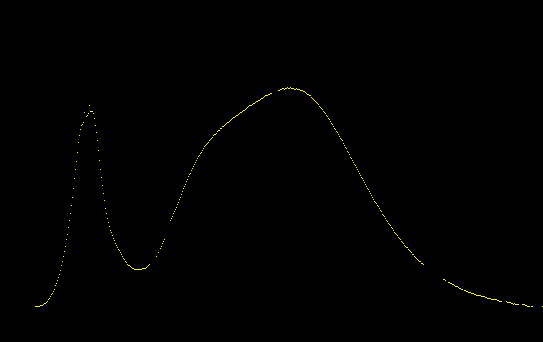## Cube root of the multiples ( cube_root(y1 * y2 * y3) )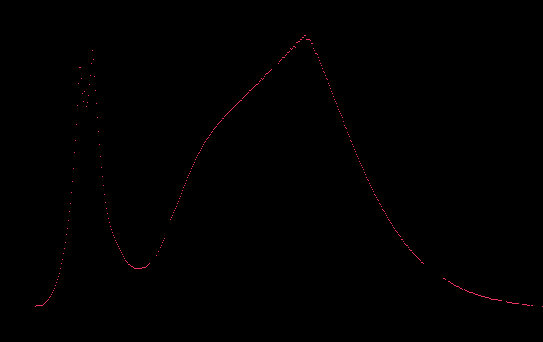## Graph of added (yellow) and multiplied (red) lines, both were scaled down.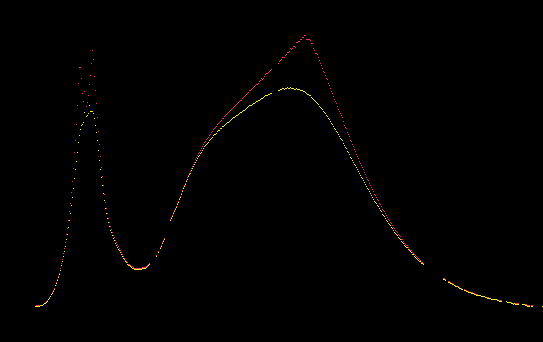## So to sum things up, these were in question## And what I thought might have looked similar after some computation.